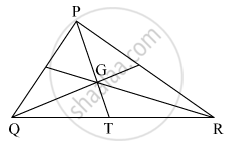Advertisement Remove all ads

# In the Given Figure, P Point G is the Point of Concurrence of the Medians of δ Pqr . If Gt = 2.5, Find the Lengths of Pg and Pt. - Geometry

Sum

In the given figure, P point G is the point of concurrence of the medians of Δ  PQR . If GT = 2.5, find the lengths of PG and PT.Advertisement Remove all ads

#### Solution

The point of concurrence of medians of a triangle divides each median in the ratio 2 : 1.
Let us suppose the length of PG and GT be 2x and x.
Therefore, the value of x is 2.5
Now, PG = 2x
= 2(2.5)
= 5 units
Now, PT = PG + GT
= 5 + 2.5
= 7.5 units
Hence, the lenght of PG and GT is 5 and 7.5units respectively.

Is there an error in this question or solution?
Advertisement Remove all ads

#### APPEARS IN

Balbharati Mathematics 2 Geometry 9th Standard Maharashtra State Board
Chapter 3 Triangles
Practice Set 3.3 | Q 4 | Page 38
Advertisement Remove all ads
Advertisement Remove all ads
Share
Notifications

View all notifications

Forgot password?